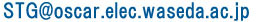STG top > Prototype STG

The Prototype Standard Task Graph Set composed of 300 task graphs, each with 50 to 2500 tasks. Prototype task graph file format is different from current Standard Task Graph Set format.

This archive is compressed by GNUtar & GNUzip. Please use these software and execute as follows:
```% tar xvzf protostg.tgz
```
then 300 task graph files named "proto???.stg" (??? = 000 to 299) are decompressed into current directory. After decompress, task graph files will occupy about 27 Megabytes.

The prototype task graph format is described below.Figure 5 shows an example prototype task graph file.
All lines
The field for each number is 6 characters wide.

First line
The "10" represents the number of tasks, and the "3" represents the number of processors to which these tasks are assigned.

Second line
The "1" represents task number 1. The "14" means that the processing time for task 1 is 14[u.t.] (Unit Time). The "0" means that task 1 has no (zero) predecessor.

Third line
The "2" represents task number 2. The "20" means that the processing time for task 2 is 20[u.t.]. The "1" means that task 2 has one predecessor. The second "1" means that the predecessor of task 2 is task 1.

Other lines
They represent the information for each task the same as above.

Last line
This line holds the information of the exit dummy node having 0[u.t.] processing time and two predecessors (task 9 and 10).
These "prototype format" is different from the Standard Task Graph Set format.

Optimal schedules of these prototype standard task graphs are shown in Optimal Schedules for Prototype Standard Task Graph Set page.Kasahara Laboratory Dept. of Electrical, Electronics and Computer Engineering, Waseda University E-Mail :